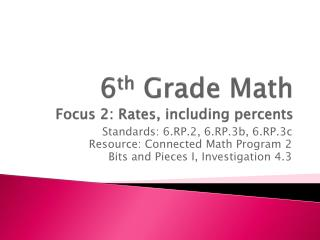DownloadDownload Presentation6 th Grade Math Focus 2: Rates, including percents

# 6 th Grade Math Focus 2: Rates, including percents

Télécharger la présentation## 6 th Grade Math Focus 2: Rates, including percents

- - - - - - - - - - - - - - - - - - - - - - - - - - - E N D - - - - - - - - - - - - - - - - - - - - - - - - - - -
##### Presentation Transcript

1. 6th Grade MathFocus 2: Rates, including percents Standards: 6.RP.2, 6.RP.3b, 6.RP.3c Resource: Connected Math Program 2 Bits and Pieces I, Investigation 4.3

2. Bits and Pieces 1- Investigation 4: Working with PercentsMathematical Learning and Problem-Solving Goals • Introduce percents as a part-whole relationship where the whole is not out of 100 but scaled to be “out of 100” (4.1) • Use fraction partitioning and fraction benchmarks to make sense of percents (4.1) • Develop strategies, including percents, to use in comparisons where the whole is less than 100 (4.2) • Understand that comparing situations with different numbers of trials is difficult unless we use percents or some other form of equivalent representation (4.2) • Work with situations where the whole is sometimes greater than 100 and sometimes less than 100 (4.3) • Develop connections between fractions, decimals, and percents (4.3) • Develop strategies for expressing data in percent form (4.3) • Relate fractions, decimals, and percents (4.4) • To move from percents to other representations and from other representations to percents (4.4)

3. Bits and Pieces 1, Investigation 4.3Mathematical Learning Targets Students will be able to work with percents by understanding and completing the following: • Work with situations where the whole is sometimes greater than 100 and sometimes less than 100 • Develop connections between fractions, decimals, and percents • Develop strategies for expressing data in percent form

4. Getting Ready for Problem 4.3

5. Think about the situations we looked at in Problems 4.1 and 4.2 • In Problem 4.1, with Yao and Shaq, we looked at a situation where the whole was GREATER than 100. • In Problem 4.2, the Portland girls’ basketball team problem, we looked at situations where the whole was LESS than 100. This problem asks you to think about fractions, decimals, and percents!

6. Problem 4.3, Parts A-D

7. TEACHER ONLY! Guide to Observations

8. TEACHER ONLY!

9. Problem 4.3, Parts E and F

10. TEACHER ONLY!

11. TEACHER ONLY! SUMMARY!

12. Summary Situation

13. Bits and Pieces 1, Investigation 4.3Mathematical Learning Targets Review Students will be able to work with percents by understanding and completing the following: • How do you work with situations where the whole is sometimes greater than 100 and sometimes less than 100? • What are some connections between fractions, decimals, and percents? • Explain a strategy you used for expressing data in percent form.

14. 6th Grade Math Homework Bits & Pieces 1 ACE #7-15

15. TEACHER ONLY!